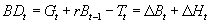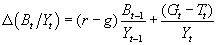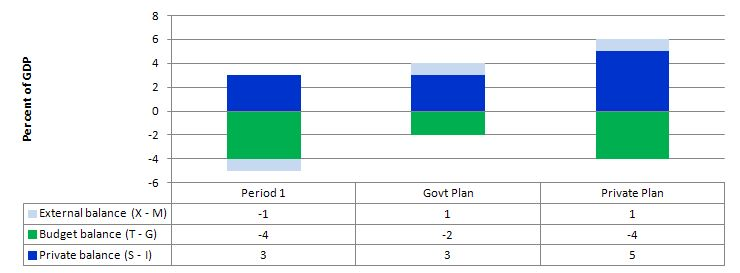# Saturday Quiz – December 14, 2013 – answers and discussion

Here are the answers with discussion for yesterday’s quiz. The information provided should help you work out why you missed a question or three! If you haven’t already done the Quiz from yesterday then have a go at it before you read the answers. I hope this helps you develop an understanding of modern monetary theory (MMT) and its application to macroeconomic thinking. Comments as usual welcome, especially if I have made an error.

Question 1:

If workers cannot maintain nominal wages growth equal to the growth in labour productivity, then their real wages fall.

The question requires you to understand what determines the real wage and what the relationship between nominal wages growth and labour productivity growth is.

The real wage is defined as the purchasing power equivalent on the nominal wage that workers get paid each period. To compute the real wage we need to consider two variables: (a) the nominal wage (W) and the aggregate price level (P).

The nominal wage (W) – that is paid by employers to workers is determined in the labour market – by the contract of employment between the worker and the employer. The price level (P) is determined in the goods market – by the interaction of total supply of output and aggregate demand for that output although there are complex models of firm price setting that use cost-plus mark-up formulas with demand just determining volume sold. We shouldn’t get into those debates here.

The inflation rate is just the continuous growth in the price level (P). A once-off adjustment in the price level is not considered by economists to constitute inflation.

The real wage (w) tells us what volume of real goods and services the nominal wage (W) will be able to command and is obviously influenced by the level of W and the price level. For a given W, the lower is P the greater the purchasing power of the nominal wage and so the higher is the real wage (w).

We write the real wage (w) as W/P. So if W = 10 and P = 1, then the real wage (w) = 10 meaning that the current wage will buy 10 units of real output. If P rose to 2 then w = 5, meaning the real wage was now cut by one-half.

The relationship between the real wage and labour productivity relates to movements in the unit costs, real unit labour costs and the wage and profit shares in national income.

The real wage will rise if nominal wages rises faster (or fall more slowly) than the price level irrespective of what is happening to labour productivity.

Labour productivity (LP) is the units of real GDP per person employed per period:

LP = GDP/L

so it tells us what real output (GDP) each labour unit (L) that is added to production produces on average. Employment here could be specified in terms of persons or person-hours, depending on the focus of the enquiry.

Unit labour costs is equal to total nominal wage costs (W times L) divided by total output:

ULC = (W x L)/GDP

You will note that this can also be written as W.(L/GDP) and L/GDP is the inverse of labour productivity. So unit labour costs rise if nominal wages growth outstrip labour productivity.

Labour productivity growth thus provides the “space” for real wages to grow without putting pressure on the price level (in a mark-up pricing world).

Now it becomes obvious that if the nominal wage (W) and the price level (P) are growing at the same pace the real wage is constant.

The following blogs may be of further interest to you:

Question 2:

Assume the central bank keeps the inflation rate steady and equal to the nominal interest rate. Under these monetary conditions, a government can push the primary budget balance into surplus and drive down the public debt ratio even if the fiscal austerity causes a recession.

The mainstream framework for analysing the so-called “financing” choices faced by a government (taxation, debt-issuance, money creation) – the government budget constraint – is written as:Which you can read in English as saying that Budget deficit = Government spending + Government interest payments – Tax receipts must equal (be “financed” by) a change in Bonds (B) and/or a change in high powered money (H). The triangle sign (delta) is just shorthand for the change in a variable.

While the mainstream textbooks think of this relationship as a financing constraint, in fact, in a stock-flow consistent macroeconomics, this relationship will always hold. That is, it has to be true if all the transactions between the government and non-government sector have been corrected added and subtracted.

So from the perspective of Modern Monetary Theory (MMT), the previous equation is just an ex post accounting identity that has to be true by definition and has no real economic importance.

For the mainstream economist, the equation represents an ex ante (before the fact) financial constraint that the government is bound by. The difference between these two conceptions is very significant and the second (mainstream) interpretation cannot be correct if governments issue fiat currency (unless they place voluntary constraints on themselves to act as if it is).

That interpretation is inapplicable when applied to a sovereign government that issues its own currency.

But the accounting relationship can be manipulated to provide an expression linking deficits and changes in the public debt ratio.

The following equation expresses the relationships above as proportions of GDP:So the change in the debt ratio is the sum of two terms on the right-hand side: (a) the difference between the real interest rate (r) and the GDP growth rate (g) times the initial debt ratio; and (b) the ratio of the primary deficit (G-T) to GDP. A primary budget balance is the difference between government spending (excluding interest rate servicing) and taxation revenue.

The real interest rate is the difference between the nominal interest rate and the inflation rate. If inflation is maintained at a rate equal to the interest rate then the real interest rate is constant.

In that case, the debt ratio will change according to the difference between the real GDP growth rate and the primary budget balance. If g = 1 (real growth 1 per cent) and the primary budget deficit was 1 per cent of GDP, then the public debt ratio would remain unchanged.

A growing economy can absorb more debt and keep the debt ratio constant or falling.

Equally, the public debt ratio can still fall even if real GDP growth is negative (recession) as long as the primary surplus is larger than the negative real GDP growth rate.

So if r = 0, and g = -1, a primary surplus equal to 2 per cent of GDP would see the public debt ratio fall by 1 per cent.

The reality is that in times of recession, a primary surplus will in all probability lead to a negative real GDP growth rate of a much larger proportion and so the public debt ratio rises, defeating the purpose of the austerity.

Similarly, a nation running a primary deficit can reduce its public debt ratio over time or hold them constant if growth is stimulated.

Further, you can see that even with a rising primary deficit, if output growth (g) is sufficiently greater than the real interest rate (r) then the debt ratio can fall from its value last period.

Depending on contributions from the external sector, a nation running a deficit will more likely create the conditions for a reduction in the public debt ratio than a nation that introduces an austerity plan aimed at running primary surpluses.

Clearly, the real growth rate has limits and that would limit the ability of a government (that voluntarily issues debt) to hold the debt ratio constant while expanding its budget deficit as a proportion of GDP.

The following blog may be of further interest to you:

Question 3:

Suppose a government announced it intended to cut its deficit from 4 per cent of GDP to 2 per cent in the coming year and during that year net exports were projected to move from a deficit of 1 per cent of GDP to a surplus of 1 per cent of GDP. If private sector deleveraging resulted in it spending less than it earned to the measure of 5 per cent of GDP, then the fiscal austerity plans will undermine growth even if the net export surplus was realised.

This question requires an understanding of the sectoral balances that can be derived from the National Accounts. But it also requires some understanding of the behavioural relationships within and between these sectors which generate the outcomes that are captured in the National Accounts and summarised by the sectoral balances.

From an accounting sense, if the external sector goes into surplus (positive net exports) there is scope for the government balance to move into surplus without compromising growth as long as the external position more than offsets any actual private domestic sector net saving.

In that sense, the government strategy in the question requires net exports adding more to aggregate demand than is destroyed by the government via its fiscal austerity. But it also implicitly assumes the private domestic sector will not undermine the strategy via increased saving overall.

Skip the next section explaining the balances if you are familiar with the derivation. The basic income-expenditure model in macroeconomics can be viewed in (at least) two ways: (a) from the perspective of the sources of spending; and (b) from the perspective of the uses of the income produced.

Bringing these two perspectives (of the same thing) together generates the sectoral balances.

From the sources perspective we write:

GDP = C + I + G + (X – M)

which says that total national income (GDP) is the sum of total final consumption spending (C), total private investment (I), total government spending (G) and net exports (X – M).

From the uses perspective, national income (GDP) can be used for:

GDP = C + S + T

which says that GDP (income) ultimately comes back to households who consume (C), save (S) or pay taxes (T) with it once all the distributions are made.

Equating these two perspectives we get:

C + S + T = GDP = C + I + G + (X – M)

So after simplification (but obeying the equation) we get the sectoral balances view of the national accounts.

(I – S) + (G – T) + (X – M) = 0

That is the three balances have to sum to zero. The sectoral balances derived are:

• The private domestic balance (I – S) – positive if in deficit, negative if in surplus.
• The Budget Deficit (G – T) – negative if in surplus, positive if in deficit.
• The Current Account balance (X – M) – positive if in surplus, negative if in deficit.

These balances are usually expressed as a per cent of GDP but that doesn’t alter the accounting rules that they sum to zero, it just means the balance to GDP ratios sum to zero.

A simplification is to add (I – S) + (X – M) and call it the non-government sector. Then you get the basic result that the government balance equals exactly \$-for-\$ (absolutely or as a per cent of GDP) the non-government balance (the sum of the private domestic and external balances).

This is also a basic rule derived from the national accounts and has to apply at all times.

If the nation is running an external surplus it means that the contribution to aggregate demand from the external sector is positive – that is net spending injection – providing a boost to domestic production and income generation.

The extent to which this allows the government to reduce its deficit and not endanger growth depends on the private domestic sector’s spending decisions (overall). If the private domestic sector runs a deficit, then the strategy will work under the assumed conditions – inasmuch as the goal is to reduce the budget deficit without compromising growth.

But this strategy would be unsustainable as it would require the private domestic sector overall to continually increase its indebtedness.

The following graph captures what might happen if the private domestic sector (households and firms) seeks to increase its overall saving at the same time the net exports are rising and the government deficit is falling.In Period 1, there is an external deficit of 1 per cent of GDP and a budget deficit of 4 per cent of GDP which generates income sufficient to allow the private domestic sector to save 3 per cent of GDP.

The Government plans to cut its deficit to 2 per cent of GDP by cutting spending. To achieve that at the same time that net exports is rising to 1 per cent of GDP then the government would be implicitly assuming that the private domestic sector would not change its saving behaviour overall.

However, what happens if the private sector, fearing the contractionary forces coming from the announced cuts in public spending and not really being in a position to assess what might happen to net exports over the coming period, decides to increase its saving. In other words, they plan to increase net saving to 5 per cent of GDP – the situation captured under the Private Plan option.

In this case, if the private sector actually succeeded in reducing its spending and increasing its saving balance to 5 per cent of GDP, the income shifts would ensure the government could not realise its planned deficit reduction.

The public and private plans are clearly not compatible and the resolution of their competing objectives would be achieved by income shifts.

In other words, as the private sector and the public sector reduced their spending in pursuit of their plans, income would contract even though net exports were rising.

The situation is that unless private sector behaviour remains constant the government cannot rely on an increase in net exports to provide the space for them to cut their own net spending.

So in general, with the government contracting the only way the private domestic sector could successfully increase its net saving is if the injection from the external sector offsett the drain from the domestic sector (public and private). Otherwise, income will decline and both the government and private domestic sector will find it difficult to reduce their net spending positions.

Take a balanced budget position, then income will decline unless the private domestic sector’s saving overall is just equal to the external surplus. If the private domestic sector tried to push its position further into surplus then the following story might unfold.

Consistent with this aspiration, households may cut back on consumption spending and save more out of disposable income. The immediate impact is that aggregate demand will fall and inventories will start to increase beyond the desired level of the firms.

The firms will soon react to the increased inventory holding costs and will start to cut back production. How quickly this happens depends on a number of factors including the pace and magnitude of the initial demand contraction. But if the households persist in trying to save more and consumption continues to lag, then soon enough the economy starts to contract – output, employment and income all fall.

The initial contraction in consumption multiplies through the expenditure system as workers who are laid off also lose income and their spending declines. This leads to further contractions.

The declining income leads to a number of consequences. Net exports improve as imports fall (less income) but the question clearly assumes that the external sector remains in deficit. Total saving actually starts to decline as income falls as does induced consumption.

So the initial discretionary decline in consumption is supplemented by the induced consumption falls driven by the multiplier process.

The decline in income then stifles firms’ investment plans – they become pessimistic of the chances of realising the output derived from augmented capacity and so aggregate demand plunges further. Both these effects push the private domestic balance further towards and eventually into surplus

With the economy in decline, tax revenue falls and welfare payments rise which push the public budget balance towards and eventually into deficit via the automatic stabilisers.

If the private sector persists in trying to increase its saving ratio then the contracting income will clearly push the budget into deficit.

So the external position has to be sufficiently strong enough to offset the domestic drains on expenditure.

The following blogs may be of further interest to you: